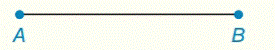Chapter 2.CR, Problem 42CRElementary Geometry For College St...

7th Edition
Alexander + 2 others
ISBN: 9781337614085

Solutions

Chapter
SectionElementary Geometry For College St...

7th Edition
Alexander + 2 others
ISBN: 9781337614085
Textbook Problem

Construct an equilateral triangle A B C with side A B ¯ .To determine

To construct:

An equilateral triangle ABC.

Explanation

Definition:

An equilateral triangle has three equal sides.

Construction:

Step (1) Consider a line AB¯ as shown in the following figure (1).

Figure (1)

Step (2) Scribe arcs from point A and B with a radius equal to the length of AB¯. These arcs intersect at point C as shown in the following figure (2).

Still sussing out bartleby?

Check out a sample textbook solution.

See a sample solution

The Solution to Your Study Problems

Bartleby provides explanations to thousands of textbook problems written by our experts, many with advanced degrees!

Get Started

(71/2)4

Applied Calculus for the Managerial, Life, and Social Sciences: A Brief Approach

83. If .

Mathematical Applications for the Management, Life, and Social Sciences

True or False: is a convergent series.

Study Guide for Stewart's Multivariable Calculus, 8th